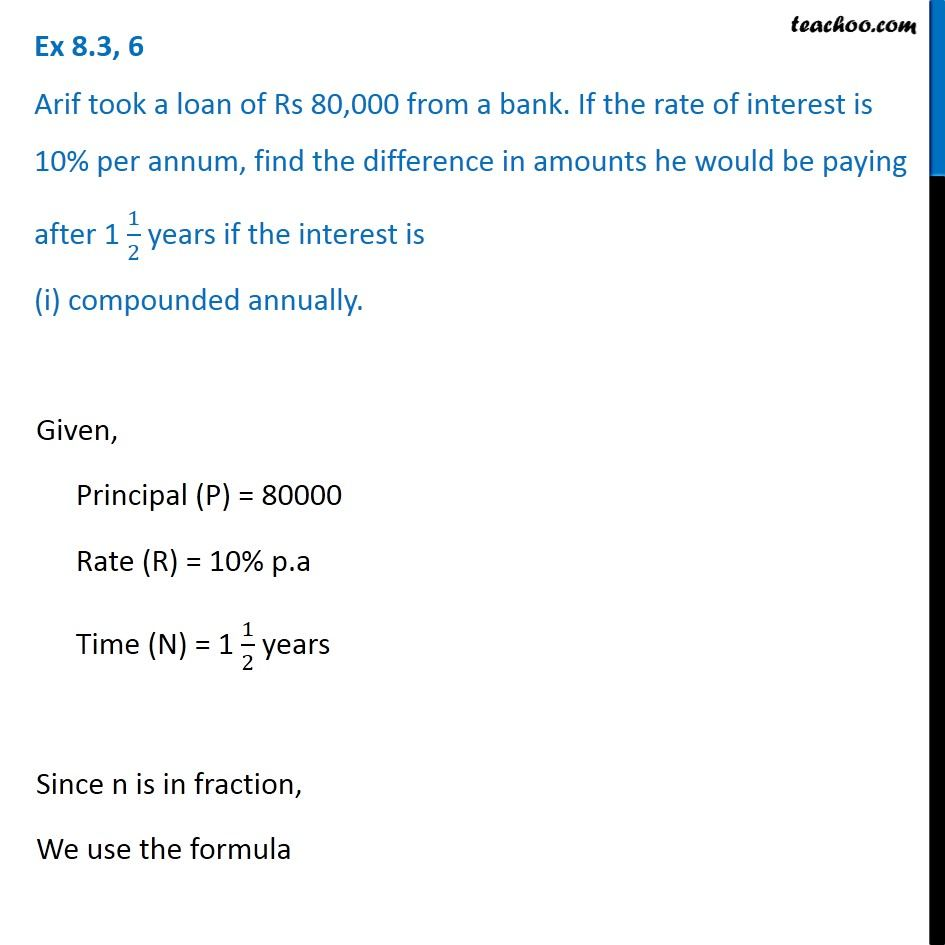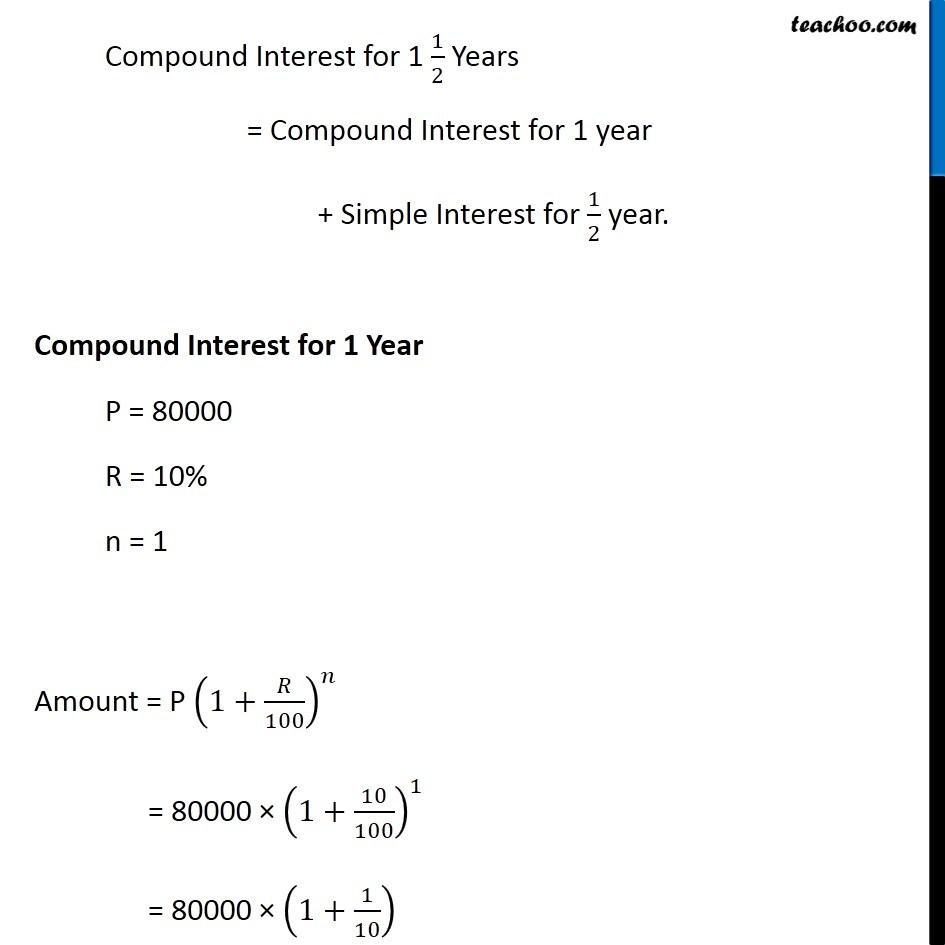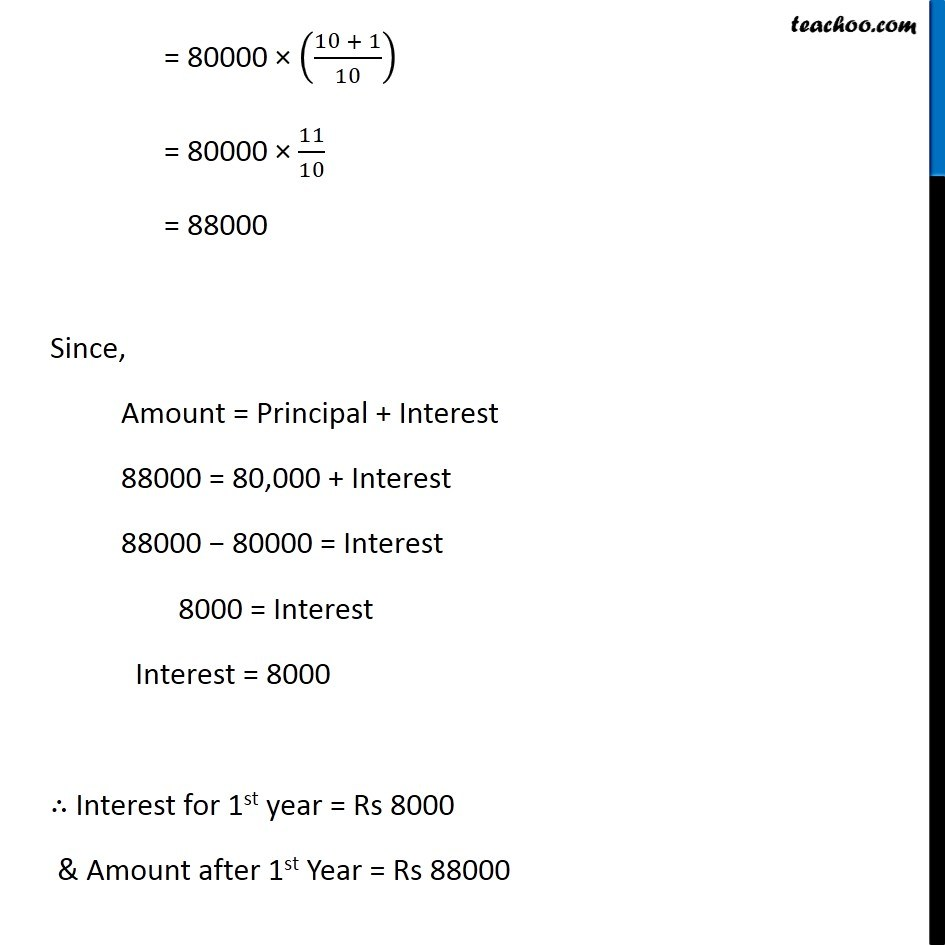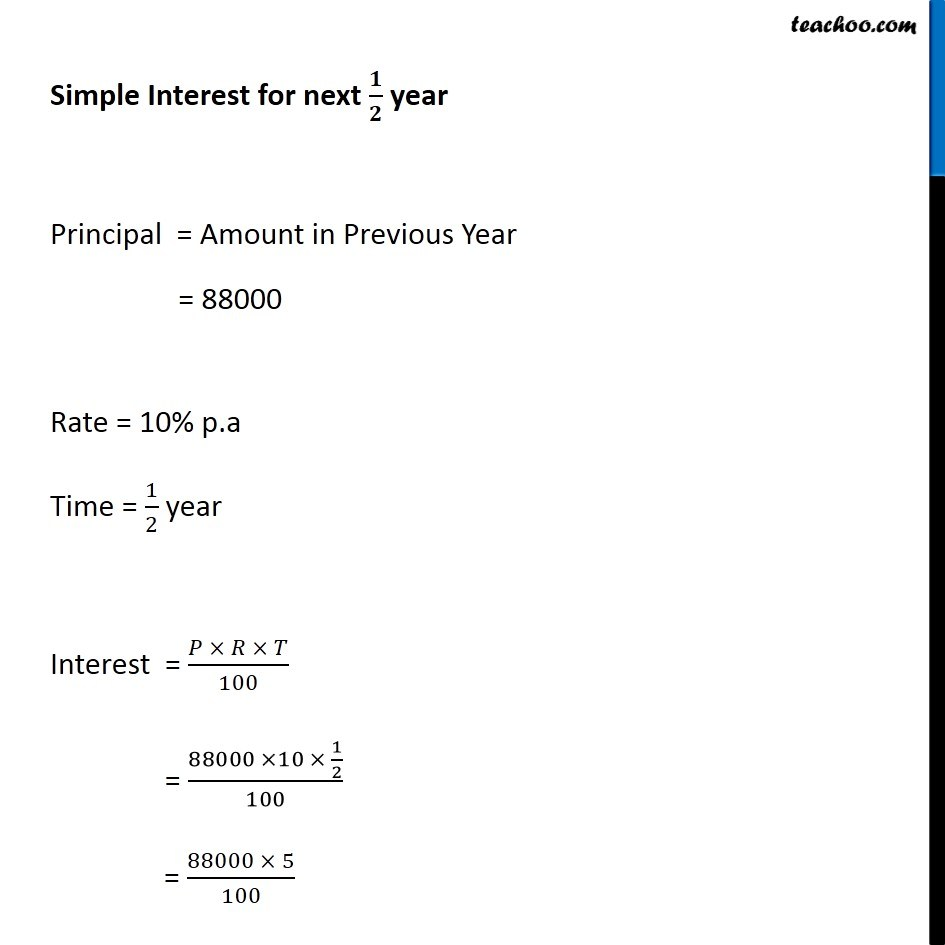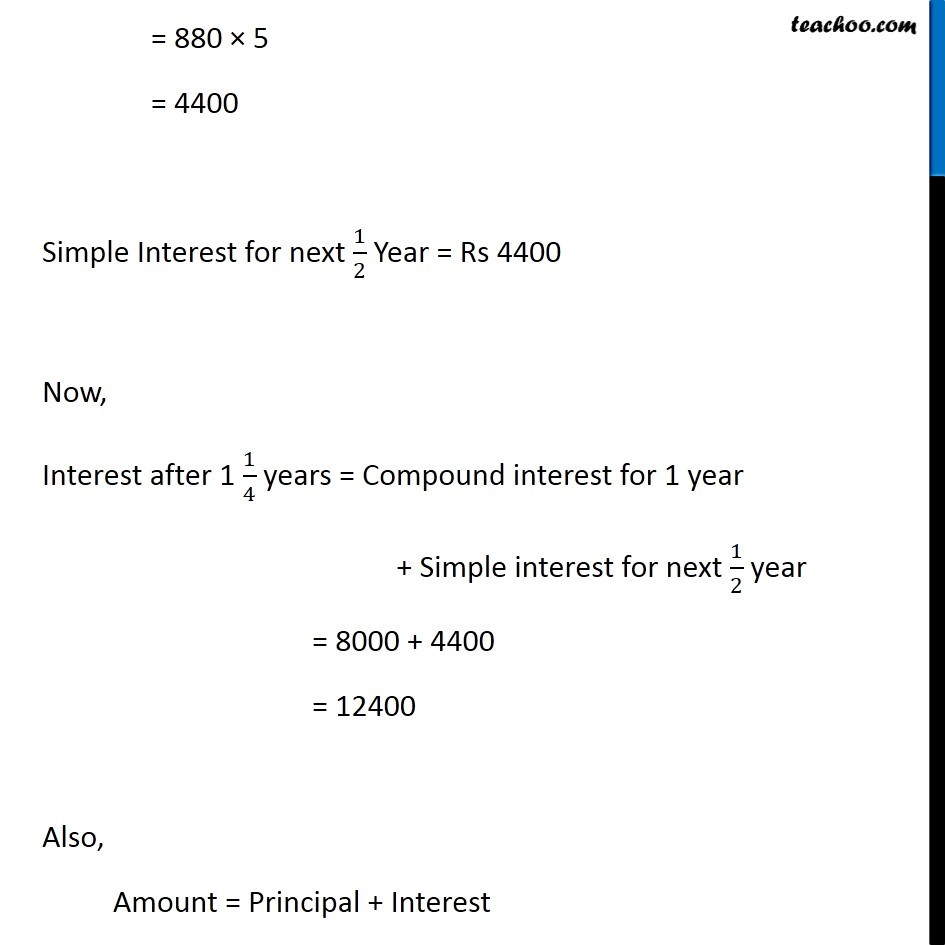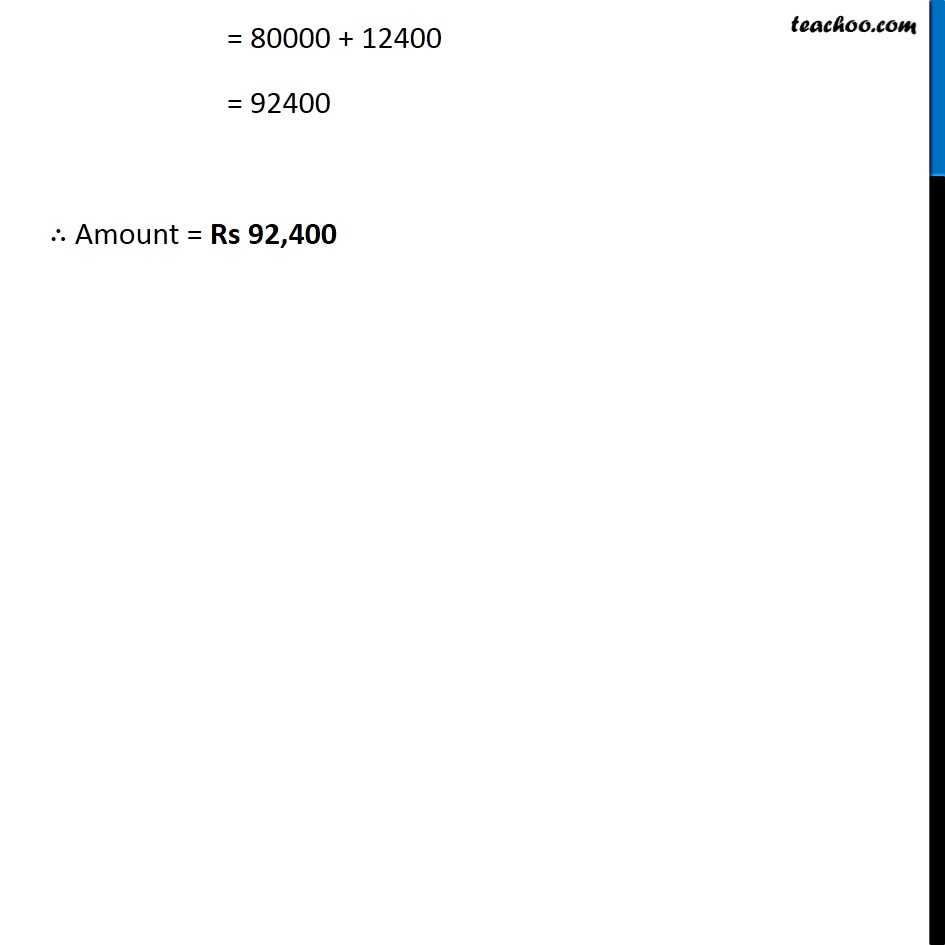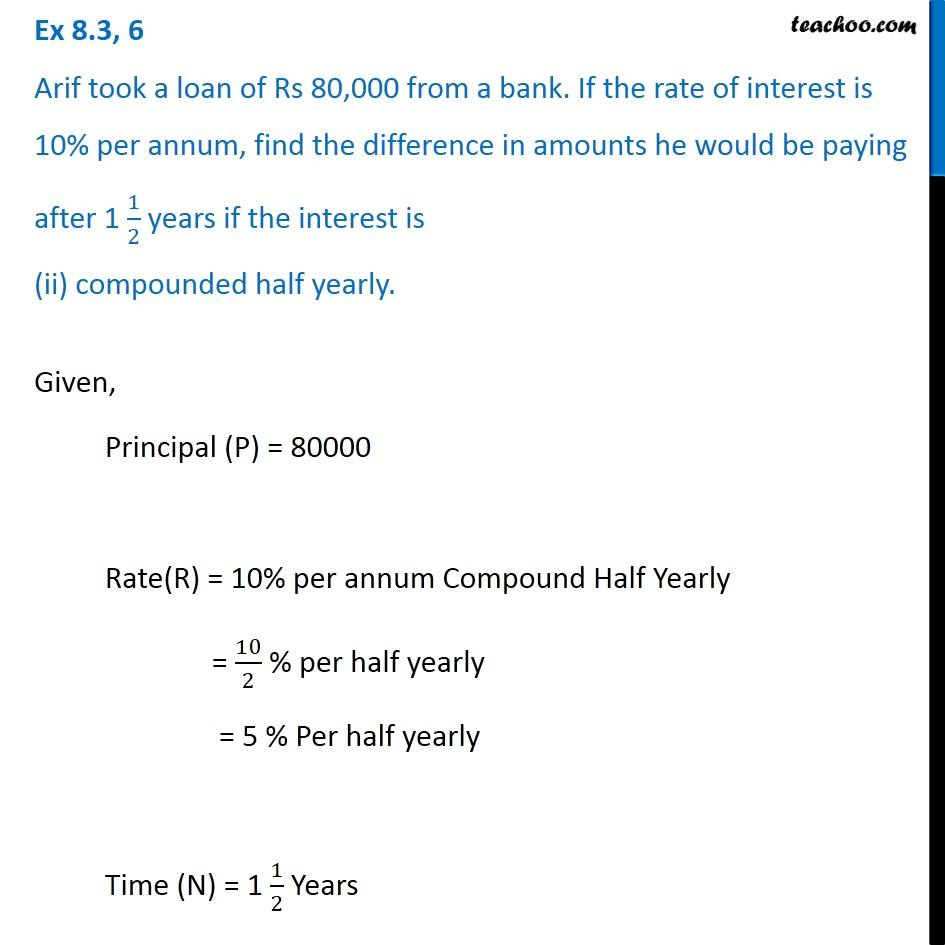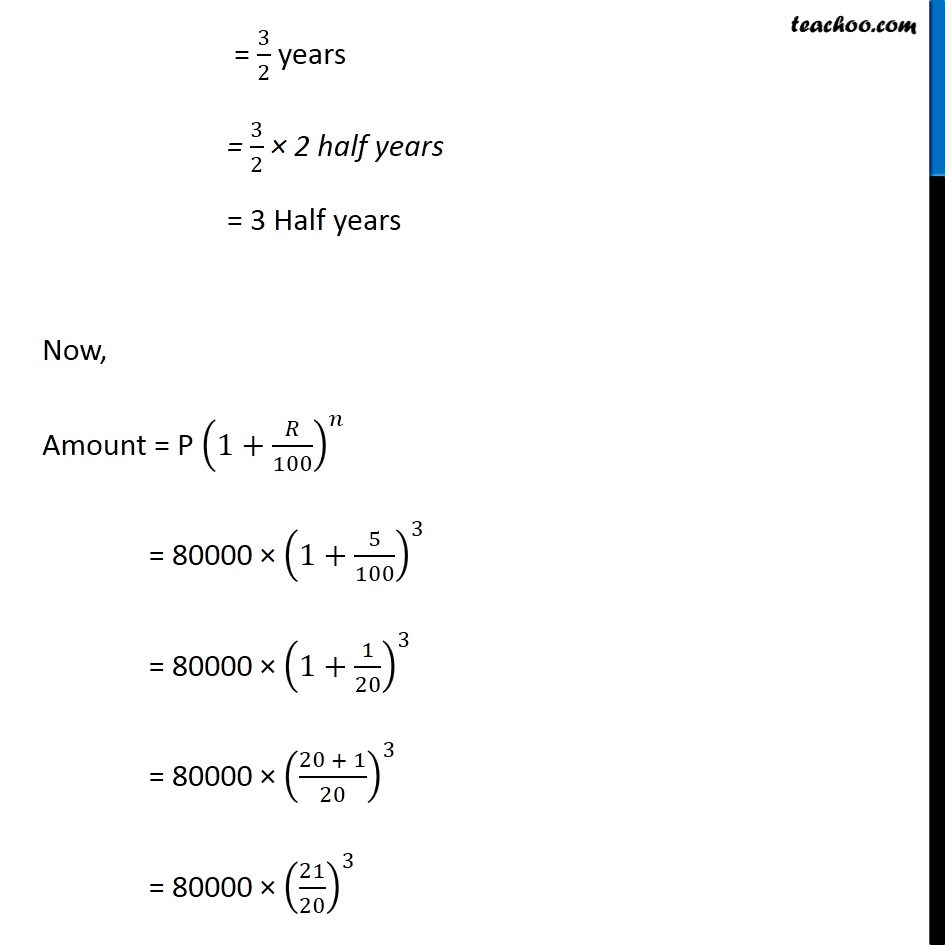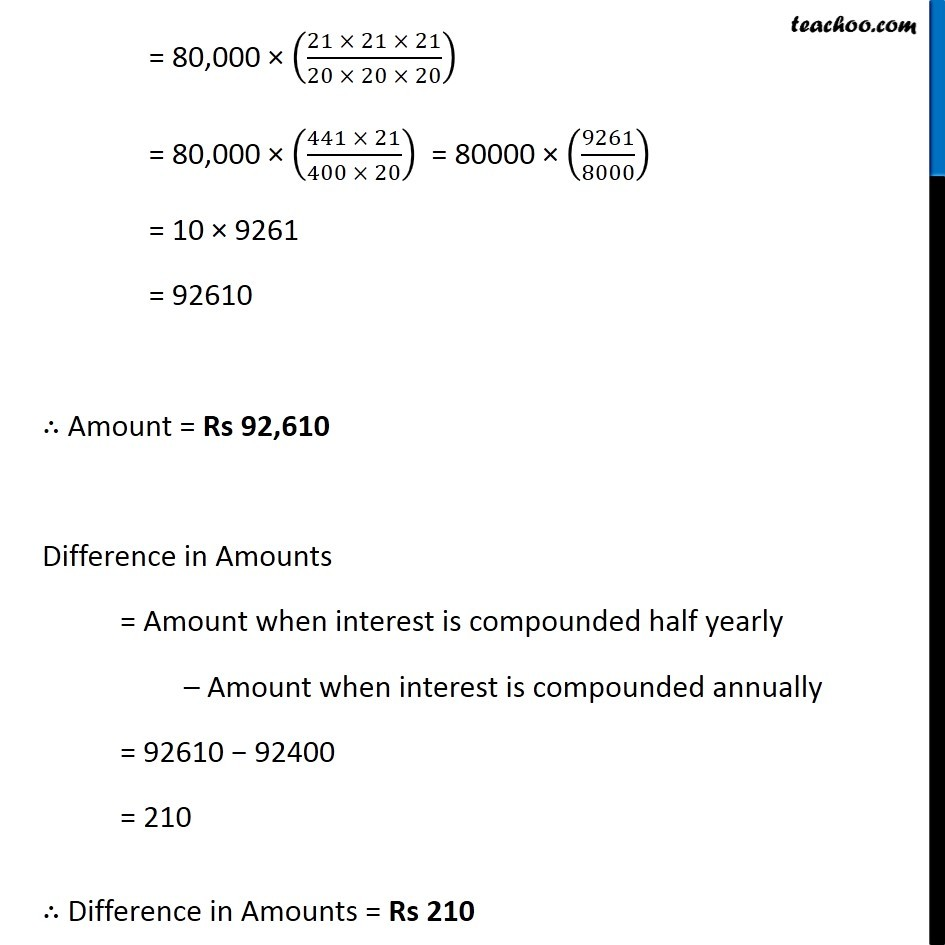Subscribe to our Youtube Channel - https://you.tube/teachoo

1. Chapter 8 Class 8 Comparing Quantities
2. Concept wise
3. Compound Interest compounded half yearly

Transcript

Ex 8.3, 6 Arif took a loan of Rs 80,000 from a bank. If the rate of interest is 10% per annum, find the difference in amounts he would be paying after 1 1/2 years if the interest is (i) compounded annually. Given, Principal (P) = 80000 Rate (R) = 10% p.a Time (N) = 1 1/2 years Since n is in fraction, We use the formula Compound Interest for 1 1/2 Years = Compound Interest for 1 year + Simple Interest for 1/2 year. Compound Interest for 1 Year P = 80000 R = 10% n = 1 Amount = P (1+𝑅/100)^𝑛 = 80000 × (1+10/100)^1 = 80000 × (1+1/10) = 80000 × ((10 + 1)/10) = 80000 × 11/10 = 88000 Since, Amount = Principal + Interest 88000 = 80,000 + Interest 88000 − 80000 = Interest 8000 = Interest Interest = 8000 ∴ Interest for 1st year = Rs 8000 & Amount after 1st Year = Rs 88000 Simple Interest for next 𝟏/𝟐 year Principal = Amount in Previous Year = 88000 Rate = 10% p.a Time = 1/2 year Interest = (𝑃 × 𝑅 × 𝑇)/100 = (88000 ×10 × 1/2)/100 = (88000 × 5)/100 = 880 × 5 = 4400 Simple Interest for next 1/2 Year = Rs 4400 Now, Interest after 1 1/4 years = Compound interest for 1 year + Simple interest for next 1/2 year = 8000 + 4400 = 12400 Also, Amount = Principal + Interest = 80000 + 12400 = 92400 ∴ Amount = Rs 92,400 Ex 8.3, 6 Arif took a loan of Rs 80,000 from a bank. If the rate of interest is 10% per annum, find the difference in amounts he would be paying after 1 1/2 years if the interest is (ii) compounded half yearly. Given, Principal (P) = 80000 Rate(R) = 10% per annum Compound Half Yearly = 10/2 % per half yearly = 5 % Per half yearly Time (N) = 1 1/2 Years = 3/2 years = 3/2 × 2 half years = 3 Half years Now, Amount = P (1+𝑅/100)^𝑛 = 80000 × (1+5/100)^3 = 80000 × (1+1/20)^3 = 80000 × ((20 + 1)/20)^3 = 80000 × (21/20)^3 = 80,000 × ((21 × 21 × 21)/(20 × 20 × 20)) = 80,000 × ((441 × 21)/(400 × 20)) = 80000 × (9261/8000) = 10 × 9261 = 92610 ∴ Amount = Rs 92,610 Difference in Amounts = Amount when interest is compounded half yearly – Amount when interest is compounded annually = 92610 − 92400 = 210 ∴ Difference in Amounts = Rs 210

Compound Interest compounded half yearly